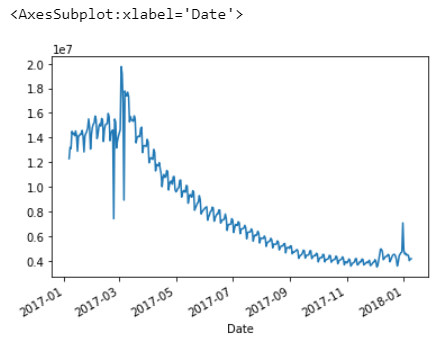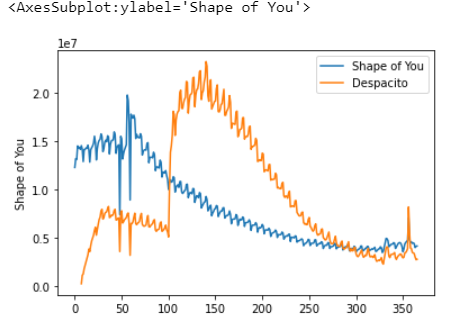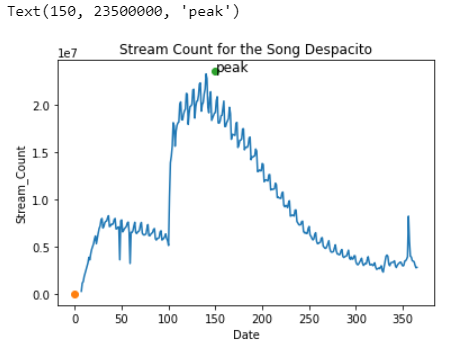Search

# Python Visualization Assignment Help | Practice Sample: 1

1- Setup the notebook

```#import Libraries
import matplotlib as mtplt #import the matplotlib library
import pandas as pd #import pandas
import matplotlib.pyplot as plt #import the scripting interface of matplotlib
%matplotlib inline  #allows to draw the plots in the same window (instead of showing the plot in a new window)
import seaborn as sns #import seaborn library```

2- Prepare the dataset

The dataset for this tutorial tracks global daily streams on the music streaming service Spotify. It focuses on five popular songs from 2017 and 2018:

• "Shape of You", by Ed Sheeran

• "Despacito", by Luis Fonzi

• "Something Just Like This", by The Chainsmokers and Coldplay

• "HUMBLE.", by Kendrick Lamar

• "Unforgettable", by French Montana

The dataset was borrowed from https://www.kaggle.com/alexisbcook/data-for-datavis?select=spotify.csv

```url = 'Spotify.csv'
df = pd.read_csv(url, index_col='Date', parse_dates= True)

Output:3- Plot the data

```df2 = df['Shape of You']
df2
df.sort_values('Date')```

Output:Plot Data

`df2.plot()`

Output:As shown in the figure above, Pandas automatically made the index (date) as the x-axis and the column "streams counts of the song" as the y-axis.

We can label the x and the y and add a title using the commands

`plt.xlabel()`
`plt.ylabel()`
`plt.title()`

```df2.plot(kind = 'line')
plt.title('Stream Count for the Song Shape of My Heart')
plt.xlabel('Date')
plt.ylabel('Stream_Count')
plt.show()```

Output:As shown the streaming spikes around March of 2017.

We use add a note in the diagram using the plt.text() method. The structure of the command is:

`plt.annotate('text', xy= (x,y),xytext= (x,y))`

x,y in this case is the x-axis and the y-axis of the marker (the spike that we want to annotate). xytext is the x-axis and y-axis of the location in which you want to add the annotation.

```df2.plot(kind = 'line')
plt.title('Stream Count for the Song Shape of My Heart')
plt.xlabel('Date')
plt.ylabel('Stream_Count')
plt.annotate('Spike', xy=('2017-03-10',17887078), xytext=('2017-03-10',17887078))
plt.show()

```

Output:Q1) Create a line plot for the Despacito song

```df2 = df['Despacito']
df2.plot(kind = 'line')
plt.title('Stream Count for the Song Despacito')
plt.xlabel('Date')
plt.ylabel('Stream_Count')
plt.show()```

Output:Q2) Create a line plot to compare the Despacito song with the Shape of You song.

```sns.lineplot(data=df['Shape of You'], label="Shape of You")
sns.lineplot(data=df['Despacito'], label="Despacito")```

Output:Q3) Add annotation to the location of the spike of the Despacito song.

```import numpy as np
df2 = df['Despacito']
df2.plot(kind = 'line')
plt.title('Stream Count for the Song Despacito')
plt.xlabel('Date')
plt.ylabel('Stream_Count')

maximum = (150,0)
minimum = (350,40)
plt.annotate("local maximum", maximum)
plt.annotate("local minimum", minimum)
plt.show()```

Output:```#text
import numpy as np
df2 = df['Despacito']
df2.plot(kind = 'line')
plt.title('Stream Count for the Song Despacito')
plt.xlabel('Date')
plt.ylabel('Stream_Count')

#annotation
plt.plot(, [0.0], 'o')
plt.plot(, [cc], 'o')
plt.annotate('peak', xy=(150, 23500000), xytext=(150, 23500000), fontsize=12)```

Output:Hire expert to get help in any visualization task, send your requirement details at realcode4you@gmail.com and get instant help with an affordable price.